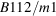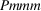International Tables for Crystallography (2016). Vol. A, ch. 1.6, pp. 107-131https://doi.org/10.1107/97809553602060000924

## Contents

• 1.6. Methods of space-group determination  (pp. 107-131)
• 1.6.1. Overview  (p. 107) | html | pdf |
• 1.6.2. Symmetry determination from single-crystal studies  (pp. 107-111) | html | pdf |
• 1.6.2.1. Symmetry information from the diffraction pattern  (pp. 107-109) | html | pdf |
• 1.6.2.2. Structure-factor statistics and crystal symmetry  (pp. 109-110) | html | pdf |
• 1.6.2.3. Symmetry information from the structure solution  (p. 110) | html | pdf |
• 1.6.2.4. Restrictions on space groups  (p. 111) | html | pdf |
• 1.6.2.5. Pitfalls in space-group determination  (p. 111) | html | pdf |
• 1.6.3. Theoretical background of reflection conditions  (pp. 112-114) | html | pdf |
• 1.6.4. Tables of reflection conditions and possible space groups  (p. 114) | html | pdf |
• 1.6.4.1. Introduction  (p. 114) | html | pdf |
• 1.6.4.2. Examples of the use of the tables  (p. 114) | html | pdf |
• 1.6.5. Specialized methods of space-group determination  (pp. 114-128) | html | pdf |
• 1.6.5.1. Applications of resonant scattering to symmetry determination  (pp. 114-126) | html | pdf |
• 1.6.5.1.1. Introduction  (pp. 114-125) | html | pdf |
• 1.6.5.1.2. Status of centrosymmetry and resonant scattering  (pp. 125-126) | html | pdf |
• 1.6.5.1.3. Resolution of noncentrosymmetric ambiguities  (p. 126) | html | pdf |
• 1.6.5.1.4. Data evaluation after structure refinement  (p. 126) | html | pdf |
• 1.6.5.2. Space-group determination in macromolecular crystallography  (pp. 126-127) | html | pdf |
• 1.6.5.3. Space-group determination from powder diffraction  (pp. 127-128) | html | pdf |
• 1.6.6. Space groups for nanocrystals by electron microscopy  (pp. 128-129) | html | pdf |
• References | html | pdf |
• Figures
• Fig. 1.6.2.1. Ideal p.d.f.'s for the equal-atom case  (p. 109) | html | pdf |
• Fig. 1.6.2.2. Exact p.d.f.'s  (p. 110) | html | pdf |
• Fig. 1.6.5.1. Data-evaluation plot for crystal Ex2  (p. 127) | html | pdf |
• Fig. 1.6.5.2. Data-evaluation plot for crystal Ex1  (p. 127) | html | pdf |
• Fig. 1.6.6.1. Polarity determination by convergent-beam electron diffraction  (p. 129) | html | pdf |
• Tables
• Table 1.6.2.1. The ability of the procedures described in Sections 1.6.2.1 and 1.6.5.1 to distinguish between space groups  (p. 108) | html | pdf |
• Table 1.6.2.2. The numerical values of several low-order moments of, based on equation (1.6.2.3)  (p. 109) | html | pdf |
• Table 1.6.3.1. Effect of lattice type on conditions for possible reflections  (p. 113) | html | pdf |
• Table 1.6.3.2. Effect of some glide reflections on conditions for possible reflections  (p. 113) | html | pdf |
• Table 1.6.3.3. Effect of some screw rotations on conditions for possible reflections  (p. 113) | html | pdf |
• Table 1.6.4.1. Summary of Tables 1.6.4.2–1.6.4.30  (p. 115) | html | pdf |
• Table 1.6.4.2. Reflection conditions and possible space groups with Bravais lattice aP and Laue class; Patterson symmetry(p. 115) | html | pdf |
• Table 1.6.4.3. Reflection conditions and possible space groups with Bravais latticeand Laue class 2/m; (monoclinic, unique axis b); Patterson symmetry(p. 115) | html | pdf |
• Table 1.6.4.4. Reflection conditions and possible space groups with Bravais lattice mS (mC, mA, mI) and Laue class 2/m (monoclinic, unique axis b); Patterson symmetry,,(p. 116) | html | pdf |
• Table 1.6.4.5. Reflection conditions and possible space groups with Bravais lattice mP and Laue class 2/m (monoclinic, unique axis c); Patterson symmetry(p. 116) | html | pdf |
• Table 1.6.4.6. Reflection conditions and possible space groups with Bravais lattice mS (mA, mB, mI) and Laue class 2/m (monoclinic, unique axis c); Patterson symmetry,,(p. 116) | html | pdf |
• Table 1.6.4.7. Reflection conditions and possible space groups with Bravais lattice oP and Laue class mmm; Patterson symmetry(pp. 117-118) | html | pdf |
• Table 1.6.4.8. Reflection conditions and possible space groups with Bravais lattice oS (oC setting) and Laue class mmm; Patterson symmetry Cmmm  (p. 119) | html | pdf |
• Table 1.6.4.9. Reflection conditions and possible space groups with Bravais lattice oS (oB setting) and Laue class mmm; Patterson symmetry Bmmm  (p. 119) | html | pdf |
• Table 1.6.4.10. Reflection conditions and possible space groups with Bravais lattice oS (oA setting) and Laue class mmm; Patterson symmetry Ammm  (p. 119) | html | pdf |
• Table 1.6.4.11. Reflection conditions and possible space groups with Bravais lattice oI and Laue class mmm; Patterson symmetry Immm  (p. 120) | html | pdf |
• Table 1.6.4.12. Reflection conditions and possible space groups with Bravais lattice oF and Laue class mmm; Patterson symmetry Fmmm  (p. 120) | html | pdf |
• Table 1.6.4.13. Reflection conditions and possible space groups with Bravais lattice tP and Laue class 4/m; hk are permutable; Patterson symmetry P4/m  (p. 120) | html | pdf |
• Table 1.6.4.14. Reflection conditions and possible space groups with Bravais lattice tP and Laue class 4/mmm; hk are permutable; Patterson symmetry P4/mmm  (p. 121) | html | pdf |
• Table 1.6.4.15. Reflection conditions and possible space groups with Bravais lattice tI and Laue class 4/m; hk are permutable; Patterson symmetry I4/m  (p. 121) | html | pdf |
• Table 1.6.4.16. Reflection conditions and possible space groups with Bravais lattice tI and Laue class 4/mmm; hk are permutable; Patterson symmetry I4/mmm  (p. 122) | html | pdf |
• Table 1.6.4.17. Reflection conditions and possible space groups with Bravais lattice hP and Laue class; hki are permutable; Patterson symmetry(p. 122) | html | pdf |
• Table 1.6.4.18. Reflection conditions and possible space groups with Bravais lattice hP and Laue classesand; hki are permutable; Patterson symmetryand(p. 122) | html | pdf |
• Table 1.6.4.19. Reflection conditions and possible space groups with Bravais lattice hP and Laue class 6/m; hki are permutable; Patterson symmetry P6/m  (p. 122) | html | pdf |
• Table 1.6.4.20. Reflection conditions and possible space groups with Bravais lattice hP and Laue class 6/mmm; hki are permutable; Patterson symmetry P6/mmm  (p. 123) | html | pdf |
• Table 1.6.4.21. Reflection conditions and possible space groups with Bravais lattice hR and Laue class(hexagonal axes); hki are permutable; Patterson symmetry; Ov = obverse setting; Rv = reverse setting  (p. 123) | html | pdf |
• Table 1.6.4.22. Reflection conditions and possible space groups with Bravais lattice hR and Laue class(hexagonal axes); hki are permutable; Patterson symmetry; Ov = obverse setting; Rv = reverse setting  (p. 123) | html | pdf |
• Table 1.6.4.23. Reflection conditions and possible space groups with Bravais lattice hR and Laue class(rhombohedral axes); hkl are permutable; Patterson symmetry(p. 123) | html | pdf |
• Table 1.6.4.24. Reflection conditions and possible space groups with Bravais lattice hR and Laue class(rhombohedral axes); hkl are permutable; Patterson symmetry(p. 123) | html | pdf |
• Table 1.6.4.25. Reflection conditions and possible space groups with Bravais lattice cP and Laue class; hkl are cyclically permutable; Patterson symmetry(p. 124) | html | pdf |
• Table 1.6.4.26. Reflection conditions and possible space groups with Bravais lattice cP and Laue class; hkl are permutable; Patterson symmetry(p. 124) | html | pdf |
• Table 1.6.4.27. Reflection conditions and possible space groups with Bravais lattice cI and Laue class; hkl are cyclically permutable; Patterson symmetry(p. 124) | html | pdf |
• Table 1.6.4.28. Reflection conditions and possible space groups with Bravais lattice cI and Laue class; hkl are permutable; Patterson symmetry(p. 124) | html | pdf |
• Table 1.6.4.29. Reflection conditions and possible space groups with Bravais lattice cF and Laue class; hkl are cyclically permutable; Patterson symmetry(p. 124) | html | pdf |
• Table 1.6.4.30. Reflection conditions and possible space groups with Bravais lattice cF and Laue class; hkl are permutable; Patterson symmetry(p. 125) | html | pdf |
• Table 1.6.5.1. Rmerge values for Ex2 for the 589 sets of general reflections of mmm which have all eight measurements in the set  (p. 126) | html | pdf |
• Table 1.6.5.2. Rmerge values for Ex1 for the 724 sets of general reflections of 2/m which have all four measurements in the set  (p. 126) | html | pdf |